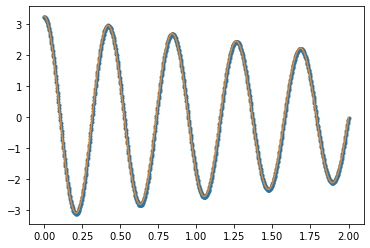# Curve Fitting¶

In the last notebook, we interactively adjusted the inertia and damping such that the simulation trajectory matched the measured data. Another way to determine the period of oscillation more accurately is to try and find the best fit curve through the data points. The Python package scipy provides a very convenient function called curve_fit. This function implements a least squares method that finds an optimal fit based on parameterized function provided by the user. Let's see how it works.

In :
import pandas as pd

In :
radial_gyro_meas = pd.read_csv('bicycle-wheel-radial-inertia-rate-gyro-measurement.csv', index_col='time')

In :
%matplotlib inline

In :
ax = radial_gyro_meas.plot(style='.')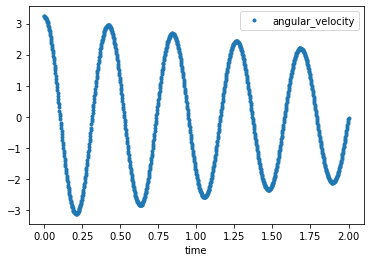## Example: Fit a cosine to the data¶

In :
import numpy as np
import matplotlib.pyplot as plt
from scipy.optimize import curve_fit
from utils import period2freq, freq2period


First define a Python function where the first input argument are the "x" values (an array) and the remaining arguments are the parameters that can be adjusted to find the optimal fit. In this case, a simple cosine function with an adjustable amplitude and frequency is created.

$$\dot{\theta} = A \cos \omega t$$
In :
def cos_func(times, amplitude, frequency):
return amplitude * np.cos(frequency * times)


To check that it works, provide some values:

In :
times = np.linspace(0, 10)
cos_func(times, 5.0, 5.0)

Out:
array([ 5.        ,  2.61509054, -2.26452058, -4.98386109, -2.94877861,
1.89933186,  4.93554853,  3.26343065, -1.52188188, -4.85537421,
-3.55701539,  1.13460728,  4.7438557 ,  3.82763759, -0.74000816,
-4.60171293, -4.07355022,  0.34063186,  4.42986349,  4.29316578,
0.06094341, -4.22941677, -4.48506653, -0.46212525,  4.00166678,
4.64801363,  0.86032381, -3.74808376, -4.78095517, -1.2529685 ,
3.47030474,  4.88303294,  1.63752457, -3.17012294, -4.95358797,
-2.01150948,  2.8494762 ,  4.99216479,  2.37250897, -2.51043448,
-4.99851436, -2.71819257,  2.15518649,  4.97259569,  3.04632869,
-1.78602555, -4.9145761 , -3.35479905,  1.4053348 ,  4.82483014])
In :
fig, ax = plt.subplots()
ax.plot(times, cos_func(times, 5.0, 5.0))

Out:
[<matplotlib.lines.Line2D at 0x7f410f07e190>]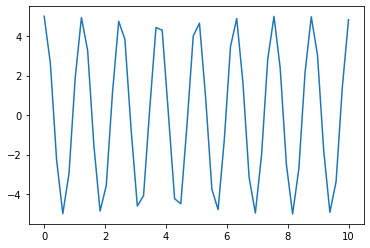In :
fig, ax = plt.subplots()

Out:
[<matplotlib.lines.Line2D at 0x7f410f058280>]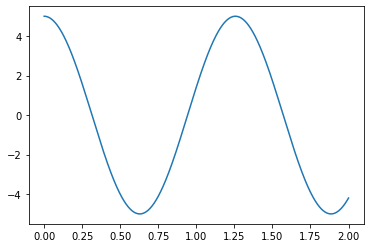Now, provide this function to curve_fit along with the measure data (x and y) and an initial guess for the amplitude and frequency. A good initial guess is important, as the optimal solution can't always be found from an arbitrary initial guess. The function curve_fit returns two items. The first is the optimal values of the two parametes and the second is the covariance matrix that gives an idea of how certain the value of the parameters are. We will just work with the first value for now.

In :
popt, pcov = curve_fit(cos_func,  # our function
p0=(3.0, period2freq(0.44)))  # the initial guess for the two parameters


Now we see the optimal values for the amplitude and frequency:

In :
popt

Out:
array([ 2.6234929 , 14.92324255])

It is useful to plot the optimal function versus the measured data:

In :
fig, ax = plt.subplots(1, 1)
ax.legend()

Out:
<matplotlib.legend.Legend at 0x7f410f03bb50>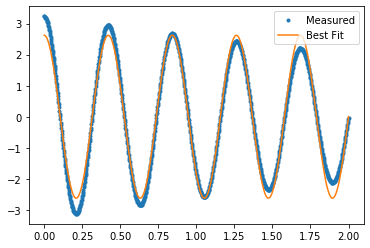That looks like a pretty nice fit! The period of this fitted function can now be computed:

In :
freq2period(popt)

Out:
0.4210335176857372

## Exercise¶

This system, just like the book on a cup system has an amplitude that decreases with respect to time. This decrease is often referred to as a decaying amplitude. We just fit the mathematical function:

$$\dot{\theta}(t) = A \cos{\omega t}$$

where $A$ is the amplitude of the angular rate $\dot{\theta}$ and $\omega$ is the frequency of oscillation in radians per second. One way to account for the decay in amplitude oscillation is to introduce a multiplicative factor of $e^{-\lambda t}$ into the equation. This will cause exponential decay at the rate $\lambda$ (units are 1 / s). The mathematical equation will look like:

$$\dot{\theta}(t) = A e^{-\lambda t} \cos{\omega t}$$

Recall that $e^{-\lambda t}$ looks like:

In :
fig, ax = plt.subplots()
t = np.linspace(0, 2, num=100)
ax.plot(t, np.exp(-1.5 * t));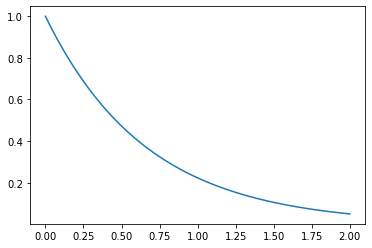Use decaying oscillation mathematical function to create a curve fitting function and find the values of $A$, $\lambda$, and $\omega$ that best fit the data. Calculate the period of oscillation and compare it to the period from the purely sinusoidal fit from above. Is there any difference in the period of oscillation?

In :
# write solution here

In :
def decaying_sinusoid(t, a, lam, w):
return a * np.exp(lam * t) * np.cos(w * t)

popt, pcov = curve_fit(decaying_sinusoid,

0.4211942223551739